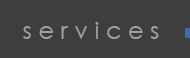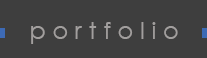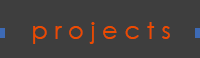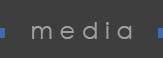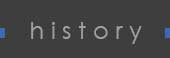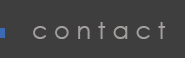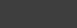S C A R S D A L E   -   S P R A G U E  (f i n a l   s t a g e s) S C A R S D A L E   -   H A T H A W A Y   (r e n o v a t i o n   i n   p r o g r e s s)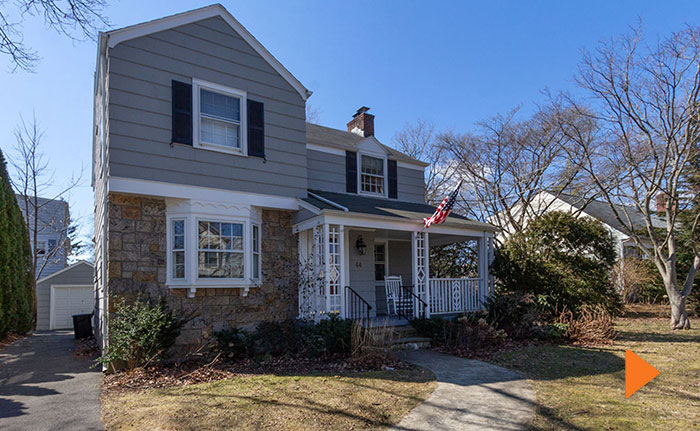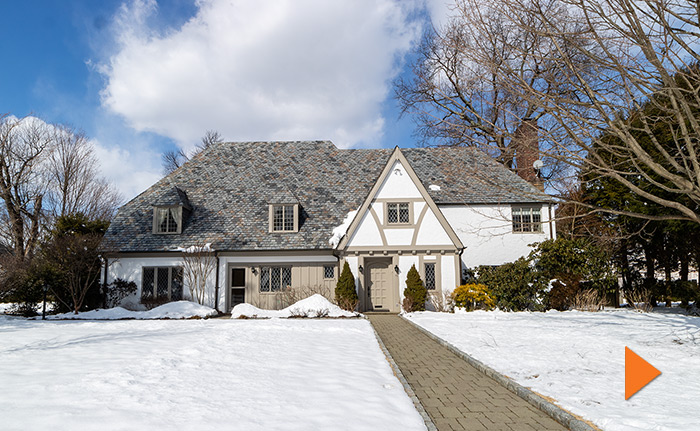S C A R S D A L E   R O U N D  H I L L    (r e n o v a t i o n   i n   p r o g r e s s) M A N H A T T A N - 3 4 T H   S T  -  O L D   C O U N T R Y   C O F F E E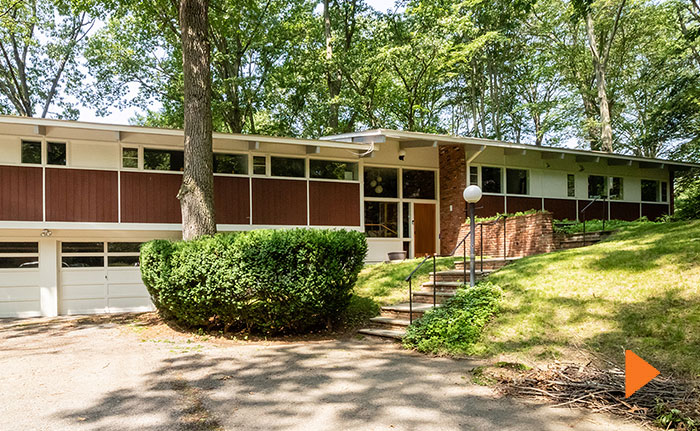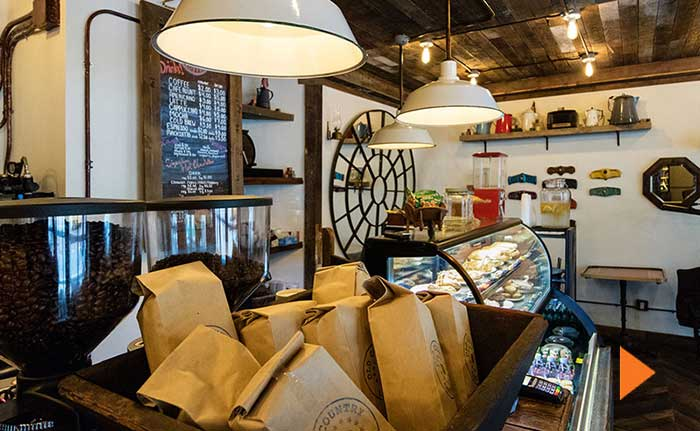Old Country Coffee is a blending of American Country and Industrial Chic styles with an Edgy Urban vibe. S C A R S D A L E  -  P O S T   R O A D P E L H A M  -   A   3 , 0 0 0   S Q   F T   M A K E O V E R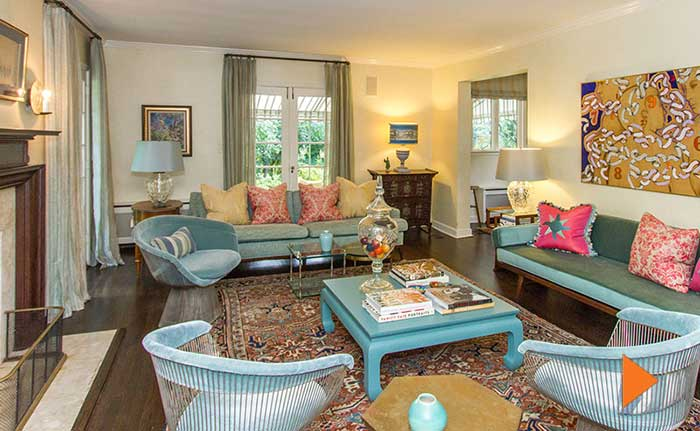The rebirth of 1144 following a catastrophic flooding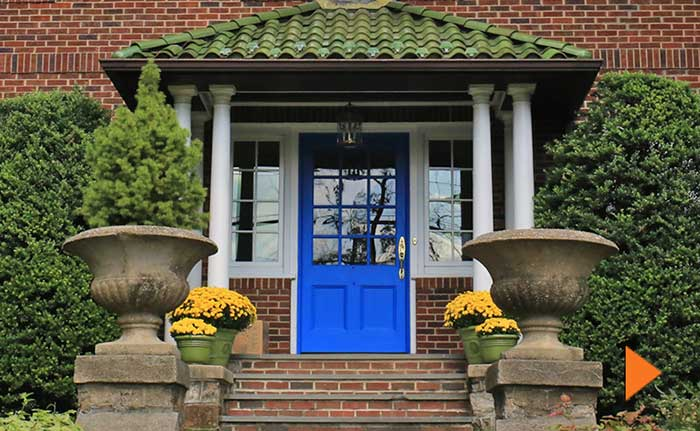Nothing will be the same as kitchen becomes bedroom and rooms get rearranged and reconfigured and rebuilt... a complete redo on this Pelham home. M A M A R O N E C K - T W I N   B A T H R O O M   R E N O V A T I O N M A N H A T T A N - 1 2 T H   S T - B A T H R O O M   F A C E L I F TC R E A T I N G   T H E   C E L E B R A T E   R O O M -  H O L I D A Y   H O U S E   2 0 1 3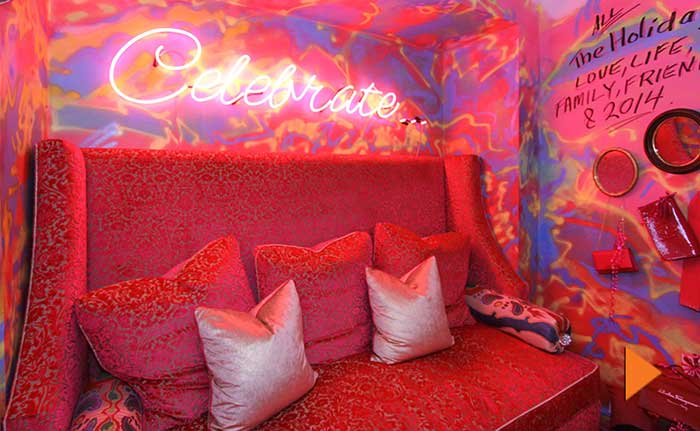The process of creating Gregory's much acclaimed pink Celebrate room, Holiday House 2013.

Copyright © 1986 - 2022 Gregory Allan Cramer          Site Design: Image Faucet - New York